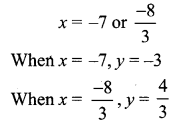You can Download Samacheer Kalvi 12th Maths Book Solutions Guide Pdf, Tamilnadu State Board help you to revise the complete Syllabus and score more marks in your examinations.

## Tamilnadu Samacheer Kalvi 12th Maths Solutions Chapter 2 Complex Numbers Ex 2.4

Question 1.
Write the following in the rectangular form:
(i) $$\overline{(5+9 i)+(2-4 i)}$$
(ii) $$\frac{10-5 i}{6+2 i}$$
(iii) $$\overline{3 i}+\frac{1}{2-i}$$
Solution: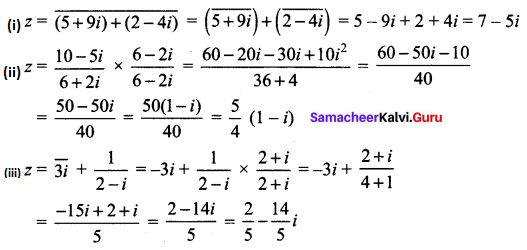Question 2.
If z = x + iy, find the following in rectangular form.
(i) Re($$\frac{1}{z}$$)
(ii) Re(i$$\bar{z}$$)
(iii) Im(3z + 4$$\bar{z}$$ – 4i)
Solution:
(i) Re($$\frac{1}{z}$$) = Re($$\frac{1}{x+i y} \times \frac{x-i y}{x-i y}$$)
= Re($$\frac{x-i y}{x^{2}+y^{2}}$$)
= $$\frac{x}{x^{2}+y^{2}}$$
(ii) Re(i$$\bar{z}$$) = Re[i($$\overline{x+i y}$$)]
= Re(ix + y)
= y
(iii) Im(3z + 4$$\bar{z}$$ – 4i)
= Im (3(x + iy) + 4(x – iy) – 4i)
= Im (3x + 3iy + 4x – 4iy – 4i)
= Im (3x + 4 + i (3y – 4y – 4)
= Im (3x + 4x + i(-y – 4))
= Im [7x + i(-y – 4)]
= -y – 4
= -(y + 4)Question 3.
If z1 = 2 – i and z2 = -4 + 3i, find the inverse of z1 z2 and $$\frac{z_{1}}{z_{2}}$$
Solution:
z1 = 2 – i, z2 = -4 + 3i
(i) z1 z2 = (2 – i) (-4 + 3i)
= (-8 + 6i + 4i – 3 i2)
= (-8 + 10i + 3)
= (-5 + 10i)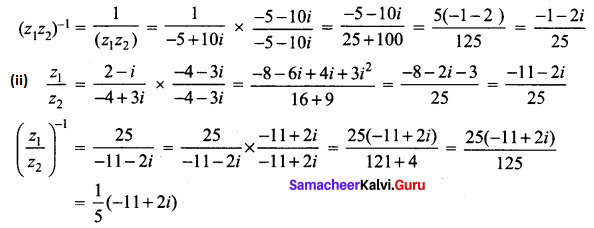Question 4.
The complex numbers u, v, and w are related by $$\frac{1}{u}=\frac{1}{v}+\frac{1}{w}$$. If v = 3 – 4i and w = 4 + 3i, find u in rectangular form.
Solution:
v = 3 – 4i, w = 4 + 3i = i (3 – 4i)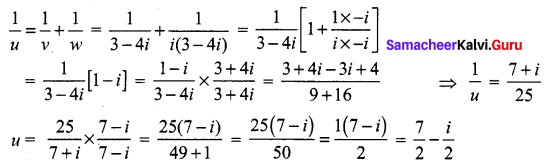Question 5.
Prove the following properties:
(i) z is real if and only if z = $$\bar{z}$$
(ii) Re(z) = $$\frac{z+\bar{z}}{2}$$ and Im(z) = $$\frac{z-\bar{z}}{2 i}$$
Solution:
(i) z is real iff z = $$\bar{z}$$
Let z = x + iy
z = $$\bar{z}$$
⇒ x + iy = x – iy
⇒ 2iy = 0
⇒ y = 0
⇒ z is real.
z is real iff z = $$\bar{z}$$
(ii) $$\frac{z+\bar{z}}{2 i}=\frac{x+i y+x-i y}{2}=\frac{2 x}{2}=x$$
Real part of z = x
(iii) $$\frac{z-\bar{z}}{2 i}=\frac{(x+i y)-(x-i y)}{2 i}=\frac{x+i y-x+i y}{2 i}=\frac{2 i y}{2 i}=y$$
Im part of z = y.

Question 6.
Find the least value of the positive integer n for which (√3 + i)n
(i) real
(ii) purely imaginary
Solution:
(√3 + i)n
(√3 + i)2
= 3 – 1 + 2√3 i
= (2 + 2 √3 i)
(√3 + i)3 = (√3 + i)2 (√3 + i)
= (2 + 2√3 i) (√3 + i)
= 2√3 + 2i + 6i – 2√3
(√3 + i) = 8i ⇒ purely Imaginary when n = 3
(√3 + i)4 = (√3 + i)3 (√3 + i)
= 8i (√3 + i)
= (-8 + 8√3 i)
(√3 + i)5 =(√3 + i)4 (√3 + i)
= (-8 + 8√3 i) (√3 + i)
= -8√3 – 8i + 24i – 8√3
= -16√3 + 16i
(√3 + i)6 = (√3 + i)5 (√3 + i)
= (√3 + i) (-16√3 + 16i)
= 16 (√3 + i) (-√3 + i)
= 16 (-3 + i√3 – i√3 – 1)
= -64 purely real when n = 6
Another Method: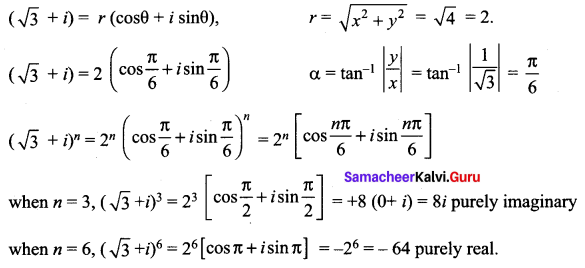Question 7.
Show that
(i) (2 + i√3)10 – (2 – i√3)10 is purely imaginary
(ii) $$\left(\frac{19-7 i}{9+i}\right)^{12}+\left(\frac{20-5 i}{7-6 i}\right)^{12}$$
Solution:
(i) (2 + i√3)10 – (2 – i√3)10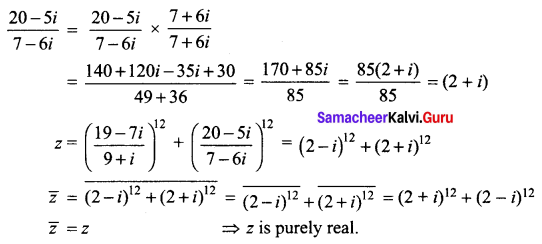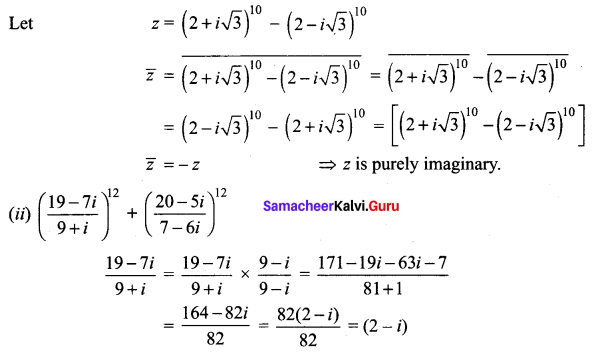### Samacheer Kalvi 12th Maths Solutions Chapter 2 Complex Numbers Ex 2.4 Additional Problems

Question 1.
Express the following in the standard form a + ib.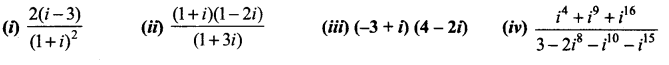Solution: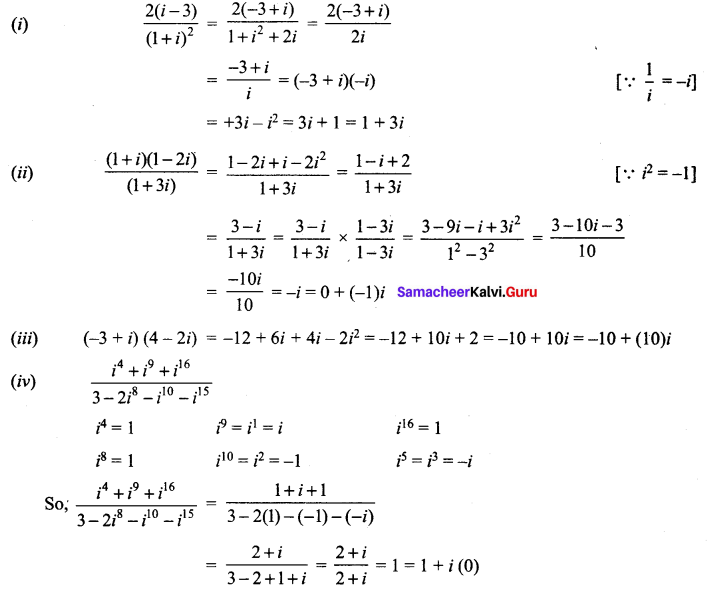Question 2.
Find the least positive integer n such that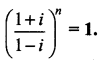Solution: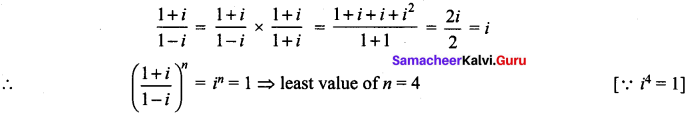Question 3.
Find the real values of x and y for which the following equations are satisfied.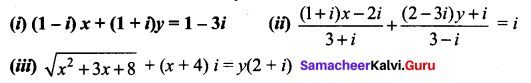Solution:
(i) (1 – i)x + (1 + i)y = x – ix + y + iy
= (x + y) + i (y – x) = 1 – 3i (given)
So, equating their RP and IP we get,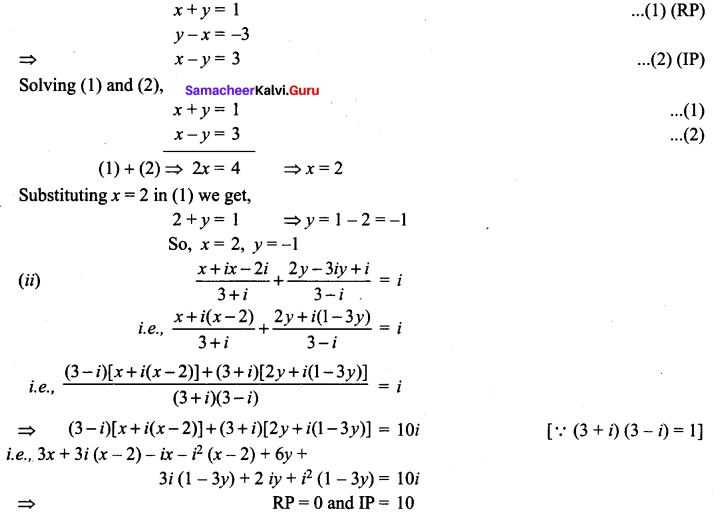Take real part, we get
i.e., 3x + (x – 2) + 6y – (1 – 3y) = 0
⇒ 3x + x – 2 + 6y – 1 + 3y = 0
4x + 9y = 3 …….. (1)
Take imaginary part, we get
3(x – 2) – x + 3 (1 – 3y) + 2y = 10
⇒ 3x – 6 – x + 3 – 9y + 2y = 10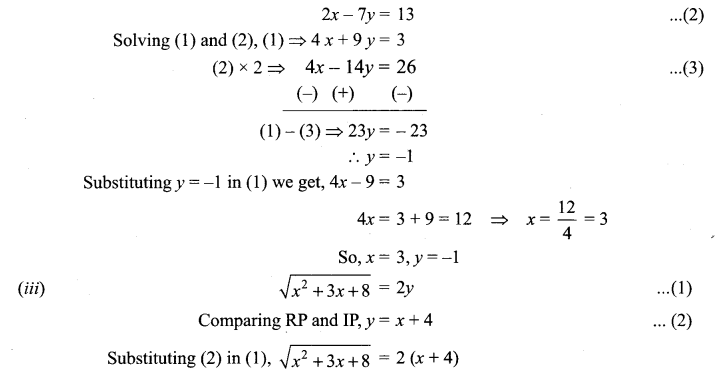Squaring on both sides, x2 + 3x + 8 = 4 (x + 4)2
i.e., x2 + 3x +8 = 4 (x2 + 8x +16) ⇒ 4x2 + 32x + 64 – x2 – 3x – 8 = 0
3x2 + 29x + 56 = 0
3x2 + 21x + 8x + 56 = 0
(x + 7) (3x + 8) = 0